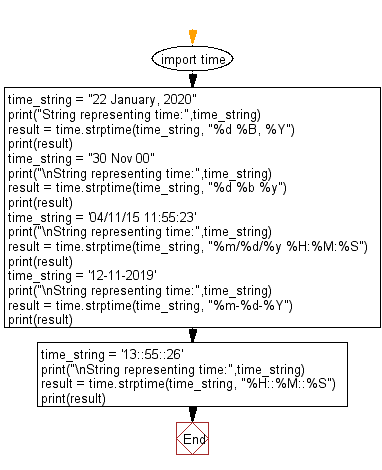﻿ Python: Parse a string representing time and returns the structure time - w3resource# Python: Parse a string representing time and returns the structure time

## Python Datetime: Exercise-63 with Solution

Write a Python program to parse a string representing time and returns the structure time.

Sample Solution:

Python Code:

``````import time
time_string = "22 January, 2020"
print("String representing time:",time_string)
result = time.strptime(time_string, "%d %B, %Y")
print(result)
time_string = "30 Nov 00"
print("\nString representing time:",time_string)
result = time.strptime(time_string, "%d %b %y")
print(result)
time_string = '04/11/15 11:55:23'
print("\nString representing time:",time_string)
result = time.strptime(time_string, "%m/%d/%y %H:%M:%S")
print(result)
time_string = '12-11-2019'
print("\nString representing time:",time_string)
result = time.strptime(time_string, "%m-%d-%Y")
print(result)
time_string = '13::55::26'
print("\nString representing time:",time_string)
result = time.strptime(time_string, "%H::%M::%S")
print(result)
```
```

Sample Output:

```String representing time: 22 January, 2020
time.struct_time(tm_year=2020, tm_mon=1, tm_mday=22, tm_hour=0, tm_min=0, tm_sec=0, tm_wday=2, tm_yday=22, tm_isdst=-1)

String representing time: 30 Nov 00
time.struct_time(tm_year=2000, tm_mon=11, tm_mday=30, tm_hour=0, tm_min=0, tm_sec=0, tm_wday=3, tm_yday=335, tm_isdst=-1)

String representing time: 04/11/15 11:55:23
time.struct_time(tm_year=2015, tm_mon=4, tm_mday=11, tm_hour=11, tm_min=55, tm_sec=23, tm_wday=5, tm_yday=101, tm_isdst=-1)

String representing time: 12-11-2019
time.struct_time(tm_year=2019, tm_mon=12, tm_mday=11, tm_hour=0, tm_min=0, tm_sec=0, tm_wday=2, tm_yday=345, tm_isdst=-1)

String representing time: 13::55::26
time.struct_time(tm_year=1900, tm_mon=1, tm_mday=1, tm_hour=13, tm_min=55, tm_sec=26, tm_wday=0, tm_yday=1, tm_isdst=-1)
```

Flowchart:## Visualize Python code execution:

The following tool visualize what the computer is doing step-by-step as it executes the said program:

Python Code Editor:

What is the difficulty level of this exercise?

Test your Programming skills with w3resource's quiz.

﻿

## Python: Tips of the Day

Understanding slice notation:

It's pretty simple really:

```a[start:stop]  # items start through stop-1
a[start:]      # items start through the rest of the array
a[:stop]       # items from the beginning through stop-1
a[:]           # a copy of the whole array
```

There is also the step value, which can be used with any of the above:

```a[start:stop:step] # start through not past stop, by step
```

The key point to remember is that the :stop value represents the first value that is not in the selected slice. So, the difference between stop and start is the number of elements selected (if step is 1, the default).

The other feature is that start or stop may be a negative number, which means it counts from the end of the array instead of the beginning. So:

```a[-1]    # last item in the array
a[-2:]   # last two items in the array
a[:-2]   # everything except the last two items
```

Similarly, step may be a negative number:

```a[::-1]    # all items in the array, reversed
a[1::-1]   # the first two items, reversed
a[:-3:-1]  # the last two items, reversed
a[-3::-1]  # everything except the last two items, reversed
```

Python is kind to the programmer if there are fewer items than you ask for. For example, if you ask for a[:-2] and a only contains one element, you get an empty list instead of an error. Sometimes you would prefer the error, so you have to be aware that this may happen.

Relation to slice() object

The slicing operator [] is actually being used in the above code with a slice() object using the : notation (which is only valid within []), i.e.:

```a[start:stop:step]
```

is equivalent to:

```a[slice(start, stop, step)]
```

Slice objects also behave slightly differently depending on the number of arguments, similarly to range(), i.e. both slice(stop) and slice(start, stop[, step]) are supported. To skip specifying a given argument, one might use None, so that e.g. a[start:] is equivalent to a[slice(start, None)] or a[::-1] is equivalent to a[slice(None, None, -1)].

While the : -based notation is very helpful for simple slicing, the explicit use of slice() objects simplifies the programmatic generation of slicing.

Ref: https://bit.ly/2MHaTp7